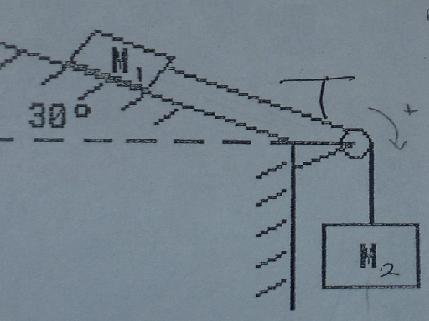# Finding Force pulling an Object Up an incline

## Homework Statement

How much force does it take to move M1 up the incline plane and what is the net force.This isnt exactly a homework equation this picture is from my last physics test where we were given M1 = 10kg, the coefficients of frictions with us = .8 and uk = .3 our job was to find m2 the acceleration and the tension. The pully is frictionless. While going over the test though our professor made told us he almost made us figure out how much force was used to lift the block M1 up and the net force once the block started moving. Ignore the arrow over the pully that was from the original problem. Im worried he will put something like this on the final.

## Homework Equations

F = ma
Fn = m1a = T - F(friction static) + W
m2a = W2 - T
a) however that is going down the slope so for going up the slope i assume i would use:
F(friction static) + Work(parallel) - T
this is where i get lost since there are two blocks it looks like the tensions would cancel out since i am using a frictionless pully and i would be subtracting the weight of block two instead of the tension. so
F(friction static) + Work(parallel m1) - W(m2) ?
If so how does that help me figure out the net once the block has started moving and is now under kinetic friction?

PhanthomJay
Homework Helper
Gold MemberHi Daishi welcome to PF!You first have to overcome static frictionto get the block to just start moving, then that force will accelerate the block once kinetic friction takes over. Apply Newton 1 for the static friction case to solve for the applied Force on M1 , then use Newton 2 to solve for acceleration when it is moving under that applied force. Note that W(parallel) is the weight component acting parallel to the plane, not the "work" done. Solve the 2 equations for T and a.

Thank you : )

Let me see if i understand what you are saying correctly. First i have to calculate for static friction which will be greater then (coefficient of static friction * mass * gravity * cos theta) (because of netwon 1st)
Then by calculating for acceleration i can use F = ma to get the force from kinetic friction (newtons 2ed)
The net would then just be the addition of the force from the kinetic plus the force of the static?

PhanthomJay
Homework Helper
Gold Member
Thank you : )

Let me see if i understand what you are saying correctly. First i have to calculate for static friction which will be greater then (coefficient of static friction * mass * gravity * cos theta) (because of netwon 1st)
Then by calculating for acceleration i can use F = ma to get the force from kinetic friction (newtons 2ed)
The net would then just be the addition of the force from the kinetic plus the force of the static?
No, this is not correct. Presumably from the original test you have already solved for the mass of block 2. Now you are being asked, as I understand the question, what is the minimum force, F, that must be applied to block 2, up and parallel to the plane, to start the block moving up the plane, and what is the net force acting on m2, once it starts moving. You will have to word the question exactly as asked.

The force required to just get the blocks to be on the verge of just about to start moving occurs when the static friction force equals usN. The static friction force cannot exceed that value. The acceleration is 0 at that point. Friction acts down the plane, the weight component of block 2 acts down the plane, and the tension on block 2 acts down the plane. The applied force acts up the plane. Now when the applied force is increased by a tiny bit, the blocks start moving, and kinetic friction takes over. Assuming the Force F is still the same, there will now be a net force acting up the plane, because the friction force is lower. Again, I am not exactly sure what the question is, since the prof probably quickly verbally asked it without an exact specific wording.

Ah, thank you very much you have been a huge help